﻿ 2-dimensional analysis of a cylindrical mixer - XSim

# 2-dimensional analysis of a cylindrical mixer

OpenFOAM 4.x

## Case directory

\$FOAM_TUTORIALS/compressible/rhoPimpleFoam/ras/mixerVessel2D

## Summary

We calculate the mixing flow as shown below with MRF function. The region "rotor" rotates at 104.72 rad/s with the Z-axis as the rotation axis, and the calculation is performed as a 2D problem with one mesh in the Z direction for 0.1 second.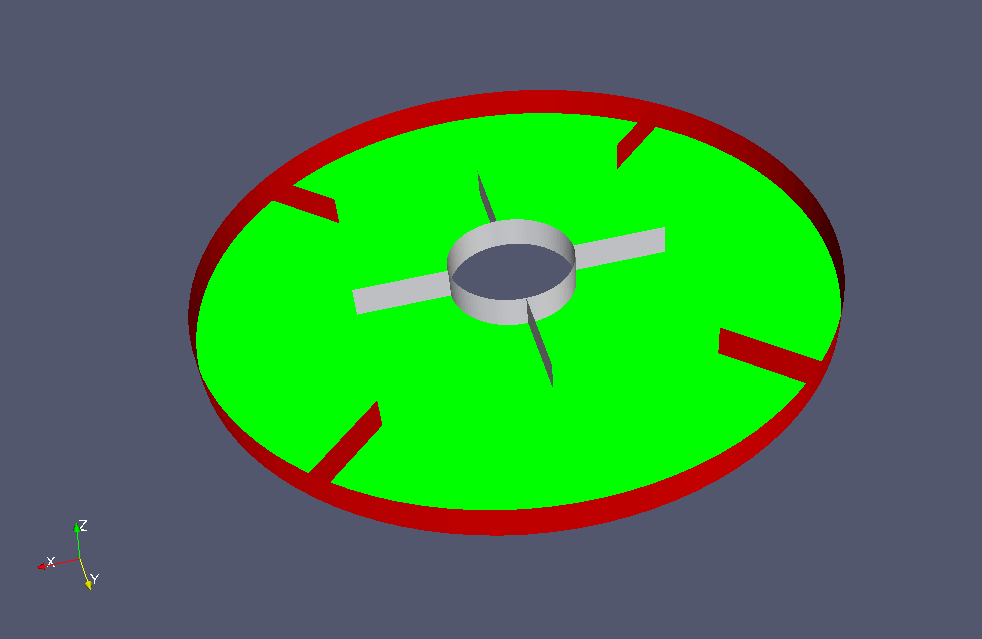Model geometry

The rotation axis and the rotation speed are defined in the file constant/MRFProperties as follows.

```MRF1
{
cellZone    rotor;
active      yes;

nonRotatingPatches ();

origin    (0 0 0);
axis      (0 0 1);
omega     104.72;
}
```

The rotation area is defined as the cell zone "rotor" as shown below (white part).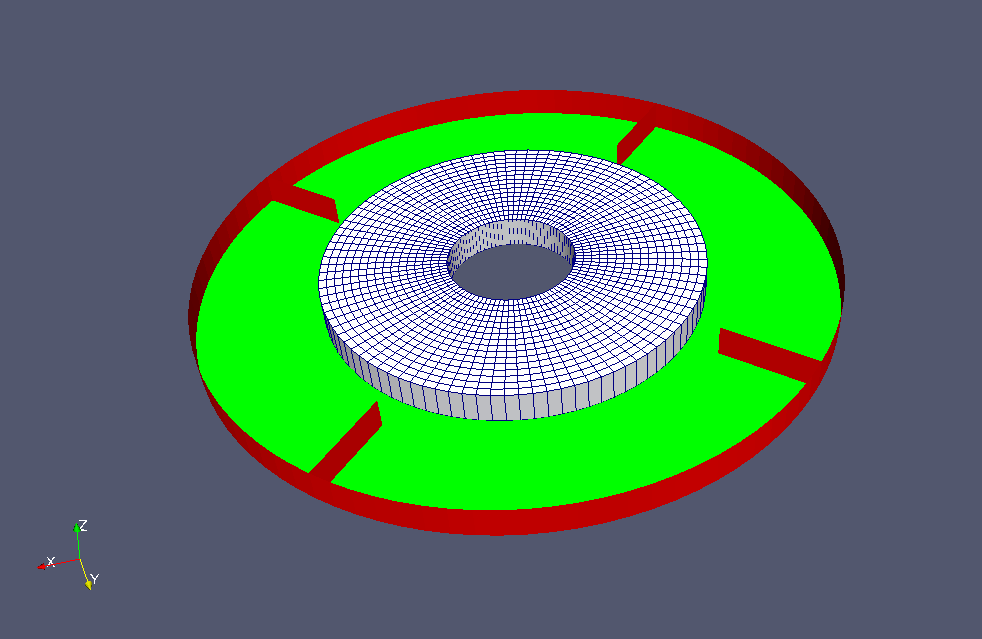cell zone "rotor"

Standard k-ε model is used as the turbulence model.

The meshes are as follows, and the number of mesh is 3072.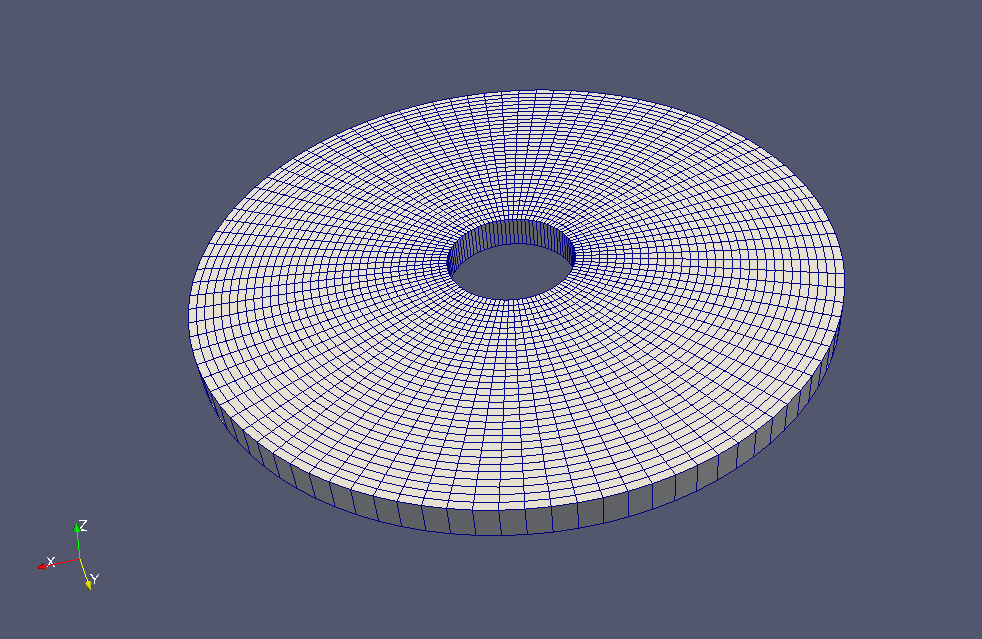Meshes

The calculation result at final time is as follows.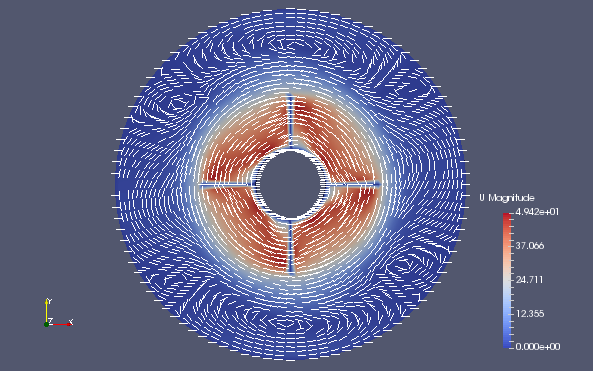Flow velocity (U)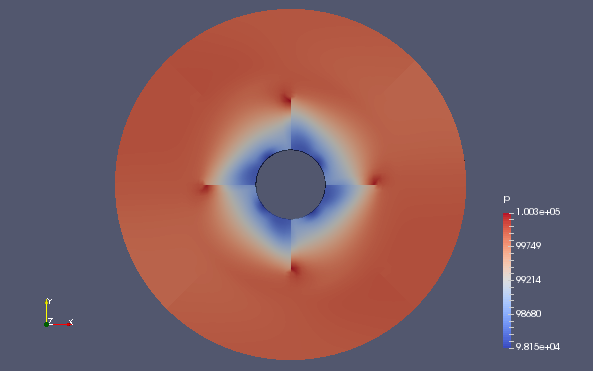Pressure (p)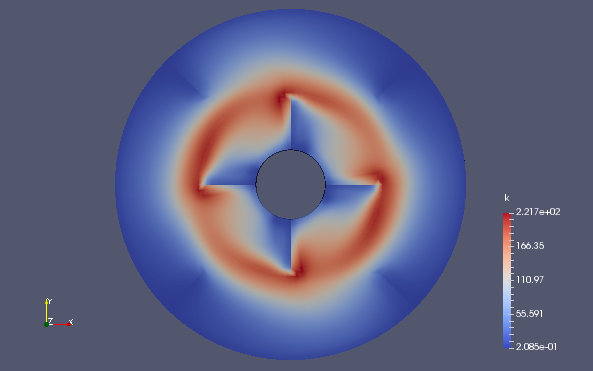Turbulent energy (k)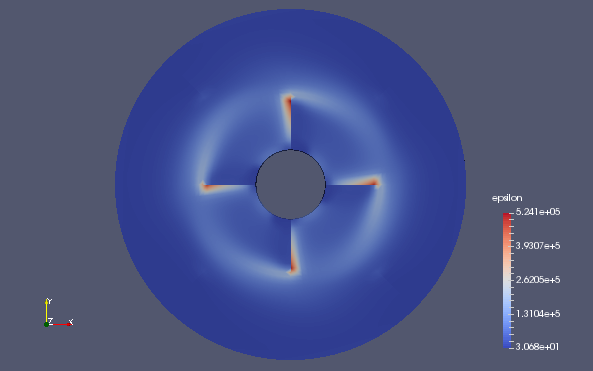Turbulent dissipation rate (epsilon)

## Commands

cp -r \$FOAM_TUTORIALS/compressible/rhoPimpleFoam/ras/mixerVessel2D mixerVessel2D
cd mixerVessel2D

m4 < system/blockMeshDict.m4 > system/blockMeshDict
blockMesh
rhoPimpleFoam

paraFoam

The macro language processor m4 is used to create the mesh. The file "system/blockMeshDict.m4" is input to m4, and the output is saved in "system/blockMeshDict". After that, blockMesh is run to create the mesh for the calculation.

## Calculation time

14.99 seconds *Single, Inter(R) Core(TM) i7-8700 CPU @ 3.20GHz 3.19GHz